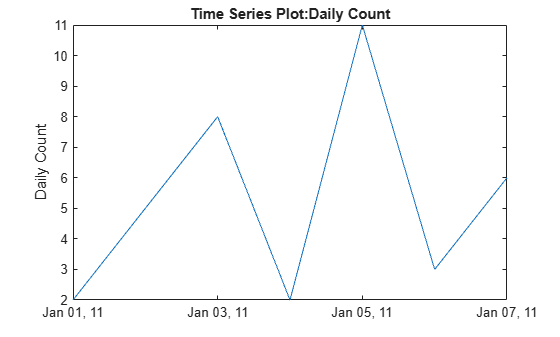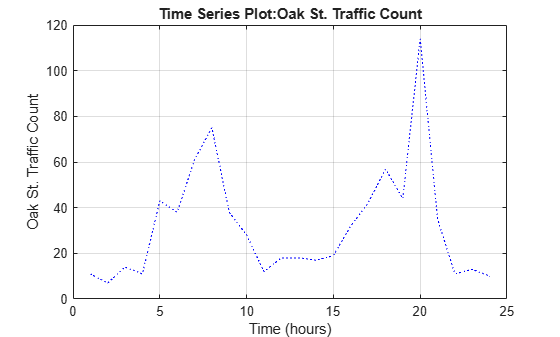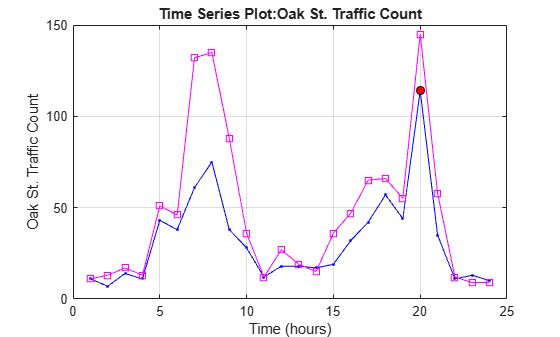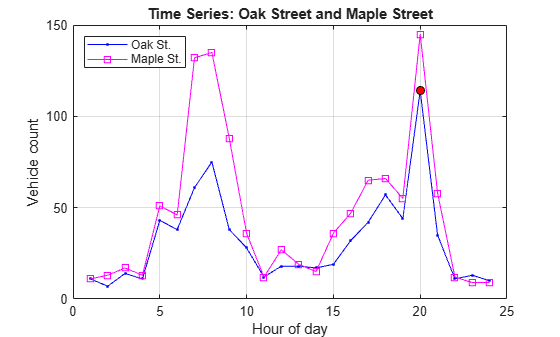# plot

Plot `timeseries`

## Syntax

``plot(ts)``
``plot(ts,specs)``

## Description

example

````plot(ts)` plots the `timeseries` data in `ts` against time, interpolating values between samples.```
````plot(ts,specs)` plots the `timeseries` data using a line graph and applies the specified `specs` to lines, markers, or both. You can also specify name-value pairs to define Line Properties.```

## Examples

collapse all

Create a time series object, set the start date, and then plot the time vector relative to the start date.

```x = [2 5 8 2 11 3 6]; ts1 = timeseries(x,1:7); ts1.Name = 'Daily Count'; ts1.TimeInfo.Units = 'days'; ts1.TimeInfo.StartDate = '01-Jan-2011'; % Set start date. ts1.TimeInfo.Format = 'mmm dd, yy'; % Set format for display on x-axis. ts1.Time = ts1.Time - ts1.Time(1); % Express time relative to the start date. plot(ts1)```Create two time series objects from traffic count data, and then plot them in sequence on the same axes. Add an event to one series, which is automatically displayed with a red marker.

```load count.dat; count1 = timeseries(count(:,1),1:24); count1.Name = 'Oak St. Traffic Count'; count1.TimeInfo.Units = 'hours'; plot(count1,':b') grid on```Obtain time of maximum value and add it as an event:

```[~,index] = max(count1.Data); max_event = tsdata.event('peak',count1.Time(index)); max_event.Units = 'hours';```

Add the event to the time series:

`count1 = addevent(count1,max_event);`

Replace plot with new one showing the event:

```plot(count1,'.-b') grid on```Make a new time series object from column 2 of the same data source:

```count2 = timeseries(count(:,2),1:24); count2.Name = 'Maple St. Traffic Count'; count2.TimeInfo.Units = 'Hours';```

Turn hold on to add the new data to the plot:

`hold on`

The plot method does not add labels to a held plot. Use property/value pairs to customize markers:

`plot(count2,'s-m','MarkerSize',6),`Labels are erased, so generate them manually:

```title('Time Series: Oak Street and Maple Street') xlabel('Hour of day') ylabel('Vehicle count')```

Add a legend in the upper left:

`legend('Oak St.','Maple St.','Location','northwest')`## Input Arguments

collapse all

Input `timeseries`, specified as a scalar.

Line specifications, specified as a character vector defining the appearance of lines, markers, or both. See `LineSpec` for more information. You can also specify name-value pairs to define Line Properties.

Data Types: `char`

## Tips

• The `plot` function generates titles and axis labels automatically. These labels are:

• Plot Title — ```'Time Series Plot: <name>'```

where `<name>` is the string assigned to `ts.Name`, or by default, `'unnamed'`

• X-Axis Label — ```'Time (<units>)'```

where `<units>` is the value of the `ts.TimeInfo.Units` field, which defaults to `'seconds'`

• Y-Axis Label — `'<name>'`

where `<name>` is the string assigned to `ts.Name`, or by default, `'unnamed'`

• You can place new time series data on a time series plot (by setting `hold on`, for example, and issuing another `timeseries/plot` command). When you add data to a plot, the title and axis labels become blank strings to avoid labeling confusion. You can add your own labels after plotting using the `title`, `xlabel`, and `ylabel` commands.

• Time series events, when defined, are marked in the plot with a circular marker with red fill. You can also specify markers for all data points using a `linespec` or name/value syntax in addition to any event markers your data defines. The event markers plot on top of the markers you define.

• The value assigned to `ts.DataInfo.Interpolation.Name` controls the type of interpolation the `plot` method uses when plotting and resampling time series data. Invoke the `timeseries` method `setinterpmethod` to change default linear interpolation to zero-order hold interpolation (staircase). This method creates a new `timeseries` object, with which you can overwrite the original one if you want. For example, to cause time series `ts` to use zero-order hold interpolation, type the following:

`ts = ts.setinterpmethod('zoh');`